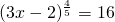# 10.2 Solving Exponential Equations

Exponential equations are often reduced by using radicals—similar to using exponents to solve for radical equations. There is one caveat, though: while odd index roots can be solved for either negative or positive values, even-powered roots can only be taken for even values, but have both positive and negative solutions. This is shown below: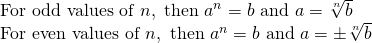Example 10.2.1

Solve for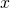in the equation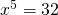.

The solution for this requires that you take the fifth root of both sides.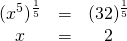When taking a positive root, there will be two solutions. For example:

Example 10.2.2

Solve forin the equation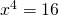.

The solution for this requires that the fourth root of both sides is taken.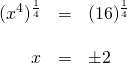The answer is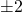because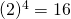and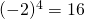.

When encountering more complicated problems that require radical solutions,  work the problem so that there is a single power to reduce as the starting point of the solution. This strategy makes for an easier solution.

Example 10.2.3

Solve forin the equation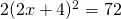.

The first step should be to isolate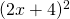, which is done by dividing both sides by 2. This results in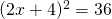.

Once isolated,  take the square root of both sides of this equation: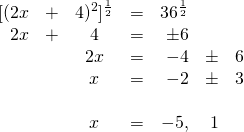Checking these solutions in the original equation indicates that both work.

Example 10.2.4

Solve forin the equation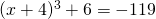.

First, isolate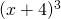by subtracting 6 from both sides. This results in.

Now,  take the cube root of both sides, which leaves: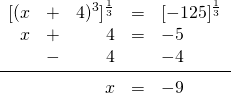Checking this solution in the original equation indicates that it is a valid solution.

Since you are solving for an odd root, there is only one solution to the cube root of −125. It is only even-powered roots that have both a positive and a negative solution.

# Questions

Solve.

1.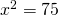2.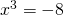3.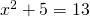4.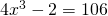5.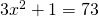6.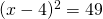7.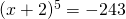8.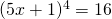9.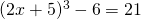10.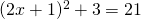11.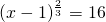12.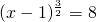13.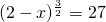14.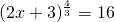15.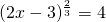16.